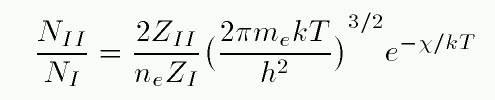# Ionization percentage in the Saha equation

tanaygupta2000
Homework Statement:
Find out the percentage of ionization in H2 gas at the centre of a star, given that the density of the star is 10^5 kg/m^3 and its temperature is 1.5 × 10^7 K.
(For H2, 2gi/ga = 1 & I = 13.6 eV).
Relevant Equations:
n2/n1 = 1/n (2πmkT/h^2)^(3/2) 2g2/g1 exp(-I/kT)
Plugging-in the above data, I am getting n2/n1 to be of the order of 10^25 !
I know that the percentage is out of 100.

Mentor
Trying to clean up your post with LaTeX...

$$n2/n1 = 1/n (2πmkT/h^2)^(3/2) 2g2/g1 exp(-I/kT)$$

Mentor
Still working on it...

Mentor
Okay, I'm not able to fix up your equation in the standard LaTeX that we use here at the PF. Please add a reply with your math properly formatted so we can try to help you. Tutorial on LaTeX is at the top of the page under INFO/Help. Thank you.

tanaygupta2000
Okay, I'm not able to fix up your equation in the standard LaTeX that we use here at the PF. Please add a reply with your math properly formatted so we can try to help you. Tutorial on LaTeX is at the top of the page under INFO/Help. Thank you.tanaygupta2000
Sir I am getting NII/NI = 139.9688 × 1025
I have checked my calculation.
How do I find the 'percentage'?

tanaygupta2000
I think I am getting confused by ne (number density of free electrons) with the density of the star.
I think I need to use ne = (Density of star)/(2mp) and not directly 105.